libalmath  2.5.11.14a
Overview

It aims to control a position and/or rotation of a part of the robot in natural 3D space. There are different mathematical ways to represent the position and the orientation of a rigid body in space. ALMath offers two ways to represent a solid in 3D:

• Transform: it is one of the best way to describe a solid in space. It is composed of a rotation matrix and a translation.
• Position6D: it is a compact representation. In basic case, it could be practical. But this representation has the drawback to have gimbal lock, which is a lost of degree of freedom.

At the end of this section, we describe how to compute the transform from a position6D.

Rotation matrix In our case, a rotation matrix is a 3*3 matrix. In linear algebra, a rotation matrix is a matrix that applies a rotation in Euclidean space.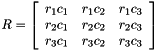with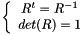The following three basic rotation matrices rotate vectors around x, y or z axis, in three dimensions.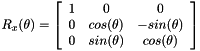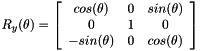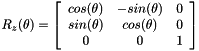Transform: Homogeneous representation

The homogenous representation is a 4*4 matrix which is composed of rotation and translation part. It represent a solid in 3D.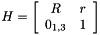with:

• R: rotation part
• r =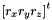: translation part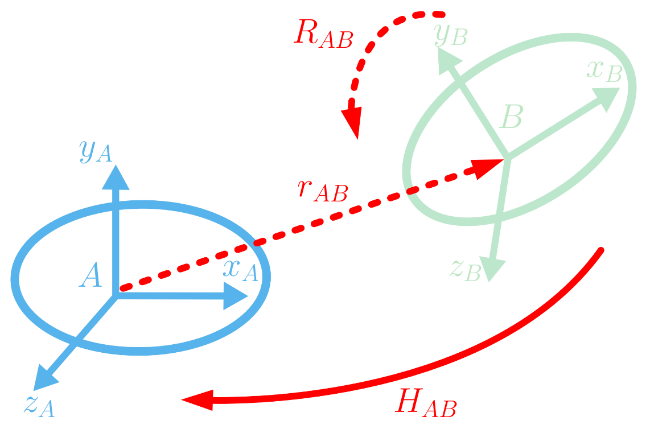Change Reference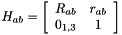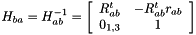Position6D

A Position6D is a vector of 6 dimensions composed of 3 translations (in meters) and 3 rotation (in radians). The angle convention of Position6D is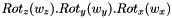.with:

•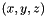: translation part (3 scalars)
•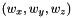: rotation part (3 scalars)

Position6D versus Transform

The following equation shows how to compute a transform from a position6D.=>with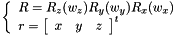Basic Example: represente a solid which position is x=1.0, y=-0.5, z=0.4 ad his orientation is 10 degree around x axis. 10 degree is equal to 0.1745 radian. In this basic case, it is very simple to use Position6D.<=>